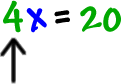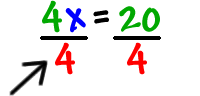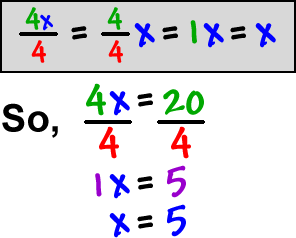We've already learned that we can add or subtract something from both sides of an equation.

So, what if we need to solve something like this?(Yep,  the answer is 5.  I know you can see it...
But, we need to learn the game.)

We need to get the x alone...We need to get this 4 out of here...

What's he doing to the x?  Multiplying!
What's the opposite of multiplying?  Dividing!

So, divide both sides by 4:Here's what's going on with this thing: